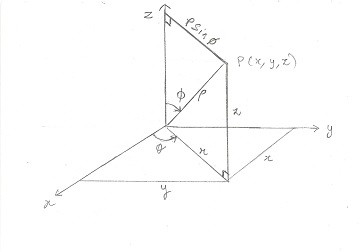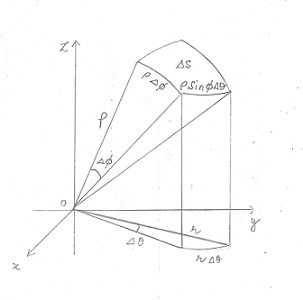# Evaluate the { \int_S (x^2z + y^2z) dS } S is the hemisphere { x^2 + y^2 + z^2 = 9, }

## Question:

Evaluate the {eq}\int_S (x^2z + y^2z) \; dS {/eq} S is the hemisphere {eq}x^2 + y^2 + z^2 = 9 {/eq}

## Surface Integrals, Spherical Coordinates:

A surface integral is evaluated across the surface area of a function representing a three dimensional surface. The surface area of a surface S is represented by the surface integral,

{eq}a(S) = \iint_S \; dS {/eq}

When the surface is spherical it is convenient to use spherical coordinates to evaluate any integral involving the surface. Rectangular coordinates can be expressed in terms of spherical coordinates as follows:

{eq}x = \rho \sin \phi \cos \theta \\ y = \rho \cos \phi \sin \theta \\ z = \rho \cos \phi {/eq}

The figure below shows the above coordinates with respect to the coordinate system.The differential element of the surface area becomes,

{eq}dS = \rho^2 \sin \phi \; d\phi \; d\theta {/eq}

If the above relations require explanations, take a look at the figure below:Become a Study.com member to unlock this answer! Create your account

The surface is spherical hence we choose spherical coordinates to parametrize the hemisphere.

The radius of the hemisphere is {eq}\rho = 3 {/eq}.

T...Cylindrical & Spherical Coordinates: Definition, Equations & Examples

from

Chapter 13 / Lesson 10
20K

Cylindrical coordinates and spherical coordinates are similar to the cartesian coordinate system but applied to specifying points in a three-dimensional plane. Learn how polar coordinates apply to these calculations and their use in celestial maps.